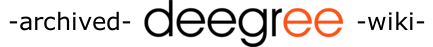[FrontPage] [TitleIndex] [WordIndex]

# Using the Rhino Javascript interpreter for testing

Here are some examples on how to use the rhino javascript interpreter that comes with recent Java versions.

## 1. Basics on using the rhino shell

You can access Java classes by specifying the complete package path

```js> var File = java.io.File
js> var f = new File("/tmp/test")
js> print(f.exists())
```

or shorter:

```js> print(java.io.File("/tmp/test").exists())
```

You can abbreviate package names and other objects (also methods!) by assigning them to variables. There is also the predefined importPackage function that imports a complete package into global scope.

```js> var utils = org.deegree.commons.utils
js> var aslist = java.util.Arrays.asList
js> utils.ArrayUtils.join("joining", aslist(["String1", "String2", "String3"]))
String1joiningString2joiningString3
js> importPackage(java.io)
```

You can use normal global state to set up your testing environment. These can also be loaded from a predefined script (using the predefined load function):

```js> var adapter = org.deegree.commons.xml.XMLAdapter
js> var url = java.net.URL
// set up a working environment
js> function refresh(){
> }
// work with the global state, reset it when necessary
js> parsed
js> refresh()
js> parsed
js> use_predefined_function()
js> // test away

```

Anonymous functions can give some extra power when it comes to code reusability. Say you need a lot of random points to test something with random geometries. Let's first set up the basics:

```var dg = org.deegree
var fac = dg.model.geometry.GeometryFactoryCreator.getInstance().getGeometryFactory()
var rnd = java.util.Random()
```

Say you want to write a function randomPoint(max, offx, offy) which generates points with values ((0-max) + offx, (0-max) + offy). Other geometries also need values like this. So you write:

```function next(max, off){
return rnd.nextDouble() * max + off
}

function randomPoint(max, offx, offy){
return fac.createPoint(null, [next(max, offx), next(max, offy)], null)
}
```

Now you have random points. But it's to expected, that the next(max, ...) calls will be plentiful, when creating line strings or polygons with more than two points. And the max parameter is always the same. So you rewrite:

```function next(max){
return function(off){
return rnd.nextDouble() * max + off
}
}

function randomPoint(max, offx, offy){
var n = next(max)
return fac.createPoint(null, [n(offx), n(offy)], null)
}
```

That way, you can use function calls to initialize local scope. In effect, you can write next(max)(off) instead of next(max, off), to achieve the same effect. The difference is that you make two function calls with one argument, instead of one function call with two arguments.

The benefit shows when testing interactively:

```function next(max){
return function(off){
return rnd.nextDouble() * max + off
}
}

function randomPoint(next, offx, offy){
return fac.createPoint(null, [next(offx), next(offy)], null)
}
js> var n = next(1000)
js> randomPoint(n, 0, 0).x
66.54616216101017
js> randomPoint(n, 100, 10).x
124.65917604565631
js> n = next(10)
js> randomPoint(n, 0, 0).x
...
```

You can customize the behaviour of your testing quite easily this way.

2018-04-20 12:04